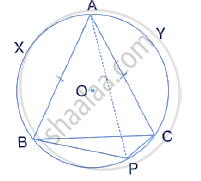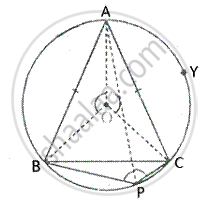Share

Books Shortlist

# In the Given Figure Abc is an Isosceles Triangle and O is the Centre of Its Circumcircle. Prove that Ap Bisects Angle Bpc . - ICSE Class 10 - Mathematics

#### Question

In the given figure ABC is an isosceles triangle and O is the centre of its circumcircle. Prove that AP bisects angle BPC .#### SolutionGiven –
∆ABC is an isosceles triangle and O is the centre of its circumcircle
To prove –
AP bisects ∠BPC
Proof –
Chord AB subtends ∠APB and chord AC subtends ∠APC at the Circumference of the circle.
But chord AB = Chord AC
∴ ∠APB = ∠APC
∴ AP is the bisector of ∠BPC

Is there an error in this question or solution?
Solution In the Given Figure Abc is an Isosceles Triangle and O is the Centre of Its Circumcircle. Prove that Ap Bisects Angle Bpc . Concept: Concept of Circles.
S